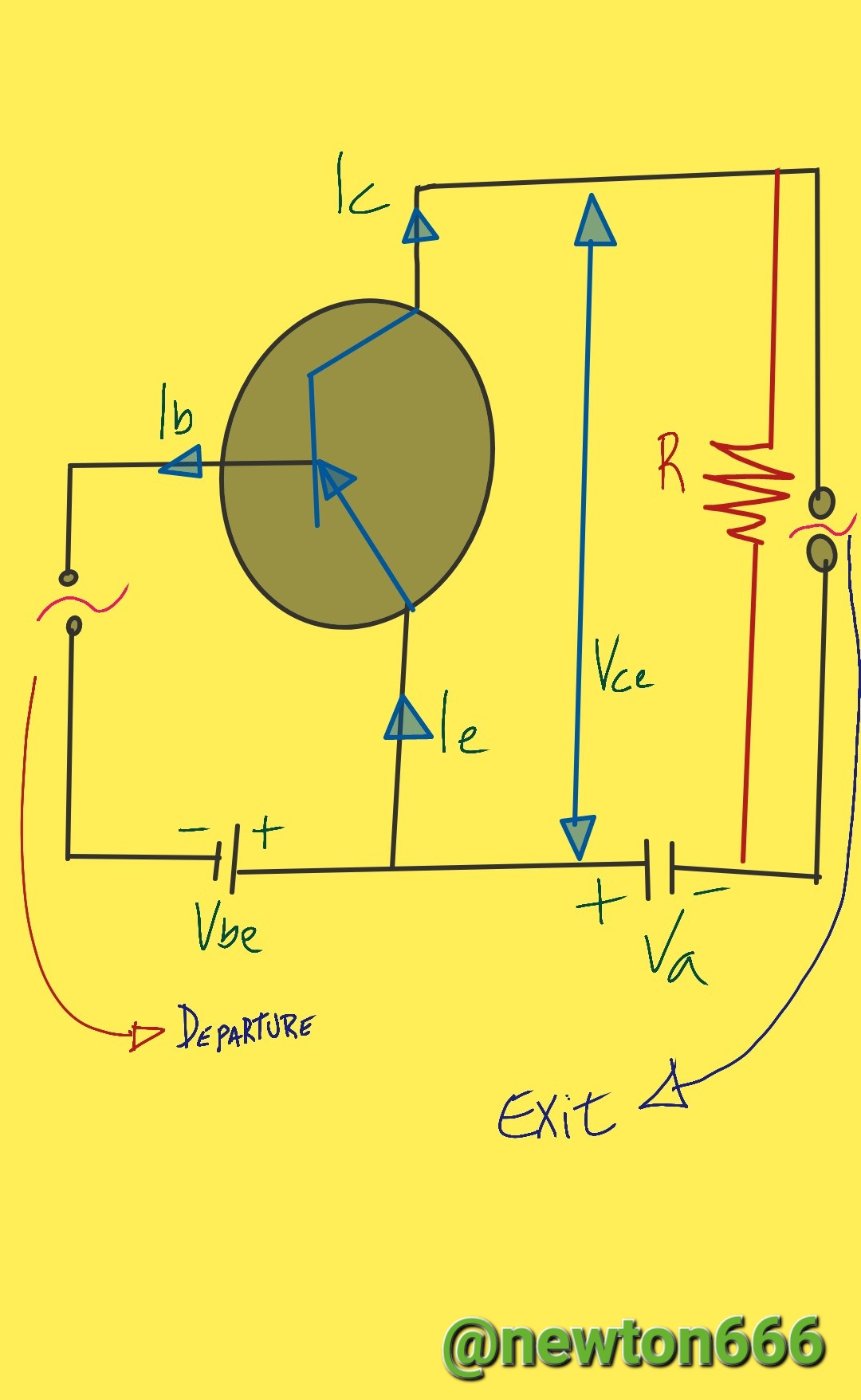# Transistor as amplifier

in StemSocial3 months ago (edited)
Hello greetings to all science lovers, this time I bring you the level of circuit physics, in my previous publication:

The section outlines the function of a transistor in a circuit, where the transistor transmits current to the collector through a positive charge power coupled to a battery at the base of the circuit. The current that flows from the collector to the emitter is controlled by the transistor's base current, and the transistor's resistance can change. Voltage is the word for the difference in electrical potential between a transistor's terminals. Depending on the ratio of the voltage at the base to the voltage at the emitter, the transistor is said to be in active mode or cutoff mode. The transistor acts as a switch in active mode, allowing a significant current to flow from the collector to the emitter.

A common electronic component found in many different circuits is the transistor. The transistor is frequently used as an amplifier in electronic circuits. The behavior of a transistor as an amplifier and the equations used to explain it are what make this special from a physics perspective.

It's crucial to comprehend the transistor's fundamental makeup before diving further into how it functions as an amplifier. The base layer, emitter layer, and collector layer are the three semiconductor layers that make up a transistor. The base layer, which lies between the emitter and the collector, is much thinner than the other two layers.

An electron current is generated at the transistor's emitter when a current is applied, and it travels through the base layer to the collector. The amount of current applied to the emitter determines how much current travels through the transistor. The transistor's ability to function as an amplifier is based on this.

An input signal is applied to the transistor's emitter to magnify a signal. The amount of current flowing from the collector to the emitter of the transistor is controlled by converting the input signal into a current that runs through the transistor. An amplified version of the input signal travels through the transistor as the output current.

The basic equation used to describe the behavior of the transistor as an amplifier is the base current equation:

Ib = (Vcc - Vbe) / Rb

Where Vcc is the power source, Vbe is the voltage drop across the transistor's base-emitter junction, and Rb is the base resistance.

The amount of current that travels from the collector to the emitter is expressed by the collector current equation:

Ic = β * Ib

Where Ic is the transistor's collector current and is its current gain.

The voltage drop across the transistor is finally described using the collector voltage equation:

Vce = Vcc - Ic * Rc

Where Vce is the voltage drop across the transistor, Rc is the collector resistance, and Vcc is the power supply.By providing an input signal to the emitter of a transistor, it can be employed as an amplifier in a circuit. The base current is used to control how much current flows through the transistor, and the output signal is amplified as a result. The base current equation, collector current equation, and collector voltage equation are some of the equations that are used to explain how a transistor behaves when acting as an amplifier. It is possible to create transistor amplifier circuits that are effective and efficient by comprehending these equations.

Photo edited by my Samsung A23 phone

Bibliography Reference

Electronics Practices by Paul B. Zbar, ‎Albert Paul Malvino, ‎Michael A. Miller, 2003.

Physics for science and technology. II by Paul Allen Tipler, ‎Gene Mosca, 2004.Sort:Thanks for your contribution to the STEMsocial community. Feel free to join us on discord to get to know the rest of us!

Please consider delegating to the @stemsocial account (85% of the curation rewards are returned).

Thanks for including @stemsocial as a beneficiary, which gives you stronger support.